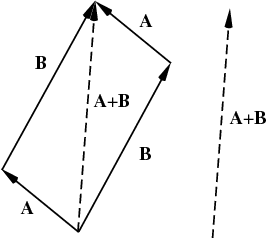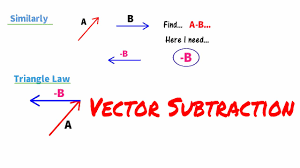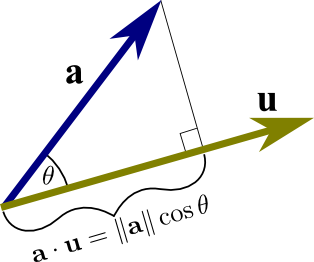Dot Product of Two Vectors

Dot Product of Two Vectors - Properties and Examples

A vector is an entity which has both magnitudes as well as direction. A vector is represented by a straight line with an arrow at one of its ends. The arrow marks the head and the other end is known as the tail of the vector. The length of the vector is known as its magnitude and the arrowhead is used to define its direction. A vector is often used to describe various entities in physics like velocities, momentum, electromagnetic fields, forces etc. In order for two vectors to be considered as same, the magnitude, as well as the direction of both the vectors, should be the same. Various algebraic operations can be applied on two vectors in space. Two vectors could be added, subtracted, and multiplied. Two vectors can be added if the tail of one vector is joined to the head of the other vector. Hence the operation is head to tail operation.Here we can see that the two vectors are added by joining head to tail. Also, one thing which we need to keep in mind is that when it comes to the addition of two vectors then the order in which we add does not matter. We can see that the addition of two vectors also full fills two important properties in mathematics, commutative and associative.

Now let us look at subtraction of two vectors. While subtracting two vectors, the vector to be subtracted from the given vector is reversed, that is, it’s direction is first reversed and then they are added as usual. When a vector is reversed then it’s sign

becomes negative. Thus, we can see thatVectors can also be multiplied. Before that let us see how vectors are represented.

A vector is represented by a letter, for example, let us take the letter a, the letter has an arrow on top of it which is used to represent its direction. The magnitude of the vector is represented by a modulus, that is, |a|. Now, before moving further we should know about a very important type of vector, the unit vector. A unit vector, for example, u, is a vector whose magnitude is unity or one. In the three dimensions, these unit vectors are represented as i, j, k in the x, y and z-direction respectively, all being in the positive direction. If we have to write these unit vectors in terms of the coordinate system then I=(1,0,0) in the positive x-direction, j=(0,1,0) in the positive y-direction and k=(0,0,1) in the positive z-direction. Similarly, we can represent any vector lying in the three dimensions if we add the multiples in the scalar form of the unit vectors, for example, let us take those scalar values as u=(u1,u2,u3) now this can be represented in the scalar form as u=u1i+u2j+u3k. In order to find the magnitude or length of this vector, we need to take the square root of the summation of the square of each of the component in the x, y, and z-direction. This can be written as |u|=sqrt(u12+u22+u32).

Now let us see how two vectors can be multiplied to each other. To multiply two given vectors, there are two methods, namely dot product and cross product. Now let us focus on the dot product of two vectors.

DOT PRODUCT OF TWO VECTORS:

In order to understand the multiplication of two vectors by the method of the dot product, we need to first understand what a projection is. A projection of one vector on the other is often referred to as the orthogonal projection of one vector on the other. Let us take two vectors, u and v, as examples. Now, if the question is asked that, how much the length of vector u is in the same direction as that of the vector v then what are we supposed to do?

Not just this, a quantity is required which will provide us any positive value if the vectors u and v will be pointing in the same direction. This quantity will provide us with a null value, that is 0 when the vector u is perpendicular to the vector v or vice versa. It will provide us with a negative value if the two vectors are lying in the opposite direction with respect to each other.
If we again look at the above question, that is, how much of the length of vector u is in the same direction as that of the vector v, then we will notice that the question is not at all about the length of the vector v. It is only related to the direction of the vector v. Now let's denote this quantity as a unit vector, that is a vector having its magnitude as unity, and also which is pointing in the same direction as that of the vector v. Therefore this vector can be represented as

a= $\frac{v}{\left | v \right |}$

Now if we multiply this resultant vector a with that of vector u with the method of dot product then we will get the answer to the above question as to how much of the vector u is lying in the direction of the vector v. This implies that u.a which is the dot product is known as the projection of the vector u on the vector a. Let us understand this with the following diagram. Here replace the vector u with vector v in the previous example that we took. The projection of the vector a in the given diagram could be seen as the perpendicular is dropped on the vector u. This forms a triangle as shown in the figure. Let us assume that the angle between the vector a and the vector u is denoted by the theta.applying the Pythagoras theorem in this triangle, we can find the formula for the dot product between the two vectors, vector a and u, in this diagram.

The formula of the dot product is

a.u= |a|cosα. Here if the angle between the two vectors is 90 degrees then we know that cos 90 is 0 so the dot product when two vectors are perpendicular to each other is zero. If the angle between them is 0 then the dot product between them will be one. If this angle is 180 degrees then the dot product will become –1, which are the vectors those are antiparallel to each other.